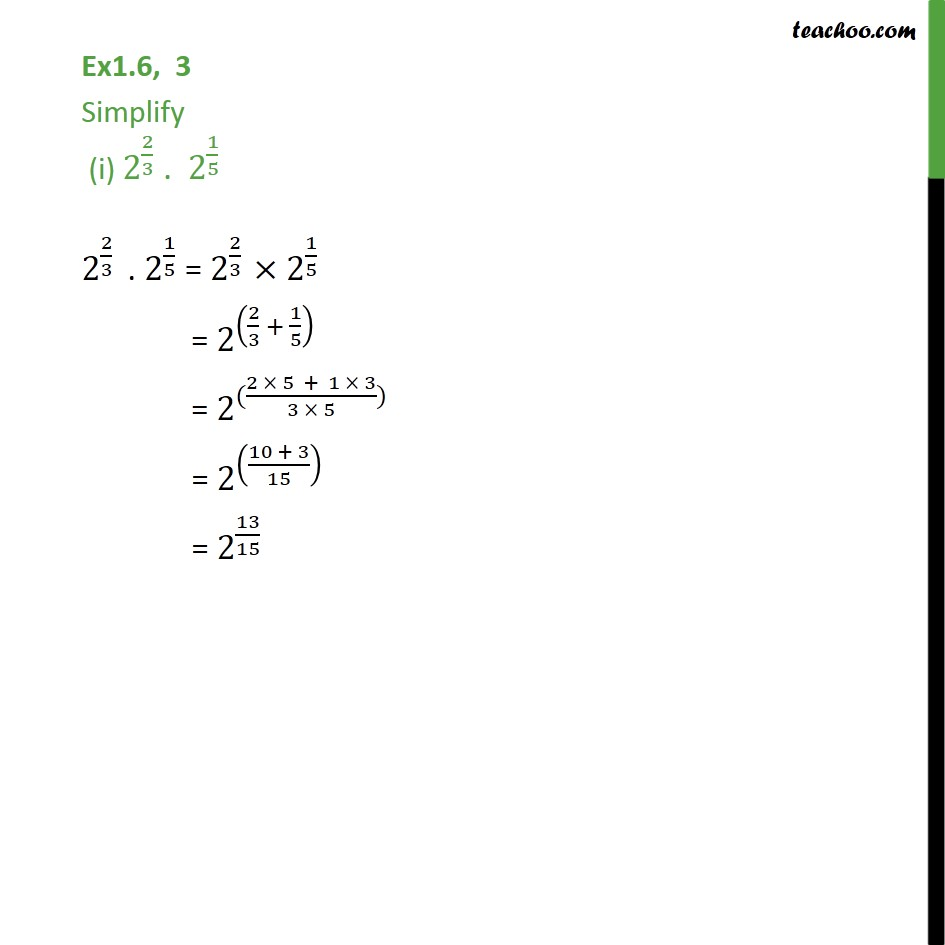1. Chapter 1 Class 9 Number Systems (Term 1)
2. Concept wise
3. Laws of exponents

Transcript

Ex1.6, 3 Simplify (i) 2^(2/3) . 2^(1/5) 2^(2/3) . 2^(1/5) = 2^(2/3) 2^(1/5) = 2^((2/3 + 1/5) ) = 2^(((2 5 + 1 3)/(3 5))) = 2^(((10 + 3)/15) ) = 2^(13/15)